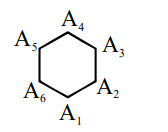# If three of the six vertices of a regular hexagon are chosen`
Question:

If three of the six vertices of a regular hexagon are chosen at random, then the probability that the triangle formed with these chosen vertices is equilateral is :

1. $\frac{3}{10}$

2. $\frac{1}{10}$

3. $\frac{3}{20}$

4. $\frac{1}{5}$

Correct Option: , 2

Solution:Only two equilateral tringles are possible $\mathrm{A}_{1} \mathrm{~A}_{3}$

$\mathrm{A}_{5}$ and $\mathrm{A}_{2} \mathrm{~A}_{5} \mathrm{~A}_{6}$

$\frac{2}{6_{C_{2}}}=\frac{2}{20}=\frac{1}{10}$